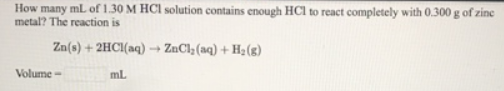# Problem: How many mL of 1.30 M HCI solution contains enough HCl to react completely with 0.300 g of zinc metal? The reaction is Zn(s) + 2HCl(aq) → ZnCl 2(aq) + H2(g)

###### FREE Expert Solution
92% (78 ratings)###### Problem Details

How many mL of 1.30 M HCI solution contains enough HCl to react completely with 0.300 g of zinc metal? The reaction is

Zn(s) + 2HCl(aq) → ZnCl 2(aq) + H2(g)